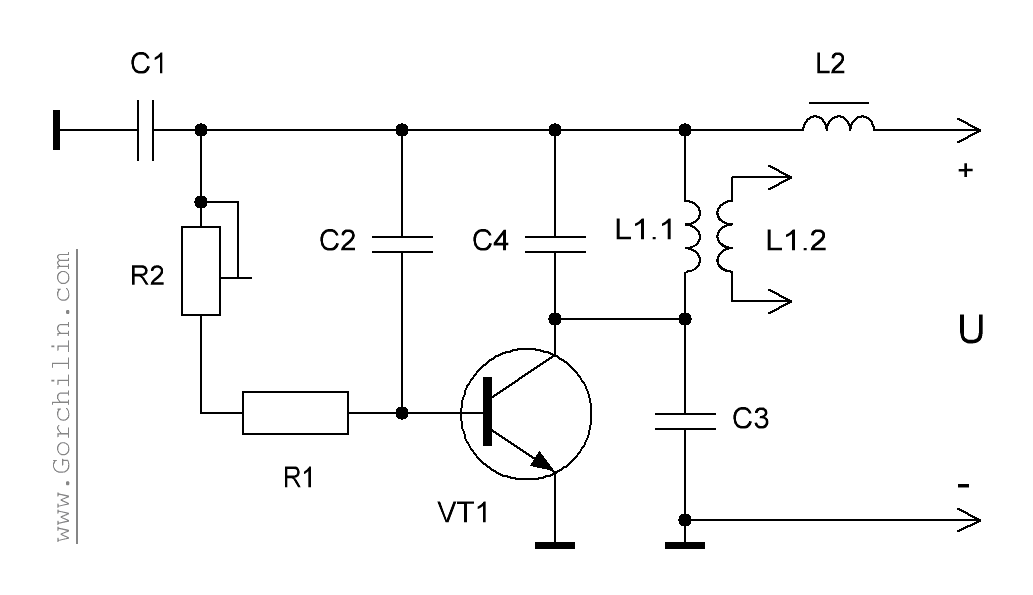Research website of Vyacheslav Gorchilin
2017-05-21
Sine wave signal generator on one transistor. Calculation
Some devices need a simple harmonic oscillator with a wide range of generated power. In this paper, we propose such a device running just a single transistor. The device allows to generate sinusoidal oscillations in a large diapazone frequency, which is determined only by the type of the transistor and to give power to the load from 0.01 to 10 watts.
Schematic diagram of the generator shown in the figure below. Resistors R1-R2 set a DC offset to the base of transistor VT1, which by means of chains of condenstion C1-C4 and inductor L1 generates the required signal. Inductor L2 is desirable but optional; its purpose is to provide ballast, in this scheme, no load will consume two to three times less power. The same load connected to the secondary winding of the coil L1. It could be a Tesla transformer (TT), reactive load, or LEDs, for example according to the scheme of treatment of the coil. By the way, if all the elements of the generator are calculated exactly, then the work of the TT in some cases it may be more efficient than the scheme katscher Brovina.The circuit can be easily controlled by external low frequency generator , to obtain at the output of the pulse packet. This can be done if the top according to the diagram R2 output file for the driver output of the generator. Another way of obtaining bundles — reduction of the resistance chain R1-R2; at a certain value, the generator switches to the so-called "fish" — bursts with a gentle buildup and decay. This mode has extremely low consumption from the power source.
Calculation
The overall calculation is defined pretty simple optimal ratios between tanks: $C_1/C_3 = 10, \quad C_2 = C_3, \quad C_3/C_4 = 5 \qquad (1.1)$ the Resonant frequency of the oscillator will be: $f_r = {1 \over 2 \pi \sqrt{L_1 C_3}} \qquad (1.2)$ the Value of resistance is determined by the gain of the transistor VT1 ($$k_{e}$$) and the voltage $$U$$, which can be from 2 to 30V depending on the type of the transistor and the required capacity. Approximate values are: $R_1 = k_{e}\,U/100 \, (k\Omega), \quad R_2 = 2 R_1 \qquad (1.3)$
Thus, the calculation begins with the value of inductance of the coil L1.1. It is possible to define the device pre-made coils, or calculated by known formulas. Please note that if TT is calculated as L1.1 there is the inductor. Calculator you can calculate the self-resonant frequency of the secondary — this frequency will need to be calculated. If calculated the usual step-up transformer, the frequency is chosen based on its parameters.
Knowing these values, we find the capacitance of the capacitor C3 from the formula (1.2): $C_3 = {1 \over (2 \pi f_r)^2 L_1} \qquad (1.4)$ And hence all other parameters: $C_1 = 10\,C_3, \quad C_2 = C_3, \quad C_4 = C_3/5 \qquad (1.5)$
Details
For small values of the supply voltage of 2-4V and power of 0.1 W is quite suitable common transistor series КТ315А or its equivalent: 2N2712, 2SC633, BFP719. For large capacities is good like transistor 2SC5200 or C4793. If the generator power up to 1W, it can not be put on the radiator.
The choke L2, you can put any standard: 100-200мкГн, for example like this. Capacitor C4 is optional. It is only necessary to correct the correction of the sine wave at the output of the generator.
The author also recommends that you read the generator voltage pulses on a single mosfet-transistor. Its circuitry is simple and it can work with any inductive load. Also, could be an interesting generator for a long line with multiple modes of operation.